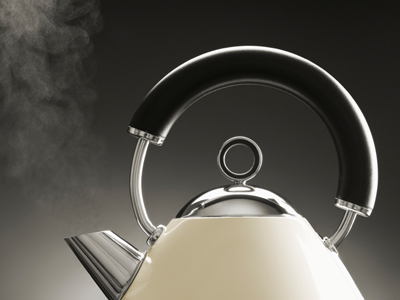If a kettle has a power rating of 5 kW and takes 3 minutes to boil some water, how much electricity has it used?

# Physics - Costing Electricity (AQA)

In GCSE Science students will spend some time looking at electrical energy. This is the second of six quizzes on that topic and it focusses on costing electricity and calculating energy transfers.

We often use electrical appliances because they transfer energy at the flick of a switch. The amount of energy transferred by the appliance depends on the power of the appliance and how long it is switched on. We can calculate how much energy is transferred by any appliance using a simple equation linking the power and the time it was switched on for:

energy transferred = power x time

You need to watch the units - if you need the energy transferred in kilowatt-hours, the power needs to be in kilowatts and the time in hours. If the power is given in watts and the time in seconds, the energy transferred will be in joules. Take care not to mix the units.

In the exams the examiners check who really understands about the correct units by perhaps giving a time in minutes. This would need converting to either a fraction of an hour or seconds, depending on what the question is asking you for. The same goes for the power. It may be given in watts but you are asked for an answer in kilowatt-hours in which case it will need converting from watts to kilowatts by dividing by 1,000 and vice versa.

From this, you can then work out how much the appliance will cost to run for the time it was on. Generally speaking, you pay for electricity measured in kilowatt-hours so questions involving cost calculations will be often be in the right units. To calculate the cost, you multiply the number of kilowatt-hours by the cost per kilowatt-hour and that is the answer. You will not be asked to convert between kilowatt-hours and joules.

We recommend that you have pen, paper and a calculator handy before starting this quiz.

1.
How much energy would be transferred by a computer of power 50 W running for 8 hours?
400 J
400 kWh
40 J
0.4 kWh
Straightforward use of the equation, but did you remember to convert the power into kilowatts (50 W = 0.05 kW)? There is no point converting the hours into seconds as a rough calculation would quickly show you that the answers in joules are nowhere near being right. If you get into the habit of doing a rough calculation in your head first, it can save errors later
2.
What is the power in watts of an electric drill that transferred 6 kWh of energy in 36 minutes?
100
1,000
5,000
10,000
Did you remember that 6 kW is 6,000 W?
3.
What does the amount of energy transferred by a television depend on?
Its power only
Its power and the length of time it is switched on
The length of time that it is switched on only
The programme that is being shown
The power consumption is often given on a label attached to the appliance
4.
In order to reduce their electricity bill, a home owner changed their electricity supplier who gave them a cheaper rate for any electricity they used between midnight and 6 a.m. This electricity cost only 8p per unit compared to 12p per unit at other times. How much would they have saved if from a total of 589 units 289 had been used at the cheaper price?
£11.56
£59.12
£23.12
£36.00
The total cost works out at £59.12 with the new supplier (£23.12 for the electricity on the cheaper rate and £36.00 for the other 300 units at the full rate)
5.
How many units of electricity would be used by a 2 kW electric fire switched on for 30 minutes?
1
2
10
60
The time in minutes needs converting to hours then you can use the equation E = P x t
6.
A consumer was told by an electricity supplier that a unit of electricity costs 10 pence. What exactly is a 'unit'?
A kilowatt-hour
A kilowatt
A joule
100 joules
When paying for electricity, you are charged for the number of kilowatt-hours you use
7.
A particular kettle has a power rating of 5 kW. It takes 3 minutes to boil enough water for a cup of tea. How much would it cost if electricity costs 10p per unit?
0.25p
2.5p
25p
75p
You need to first convert the 3 minutes to a fraction of an hour (3 ÷ 60 = 0.05) before you can work out how many units were used
8.
A home owner reads their electricity meter on January 1st and again on the last day of the month. The first value was 4,792 kWh, the second reading was 5,381 kWh. If their electricity cost 12p per unit, what was their January bill?
£7.68
£60.00
£70.68
£76.80
Take the difference between the two readings and multiply by 0.12 to get the answer in pounds
9.
How long could you run a 2 kW hairdryer on 50p if electricity costs 7.5p per unit?
Just over 3 hours
Just under 3 hours
2 1/2 hours
2 hours
The time is worked out by dividing the energy transferred by the power. Before you do that, you need to work out the energy transferred in units then divide the amount of money to be spent by the cost per unit which tells you how many kWh your 50p would buy
10.
If an appliance consumes (transfers) electrical energy at a rate of 8 kWh and electricity costs 8 pence per unit. What is the cost of running it for 3 hours?
8p
19p
64p
£1.92
8 kWh x 3 hours x 8p gives the answer